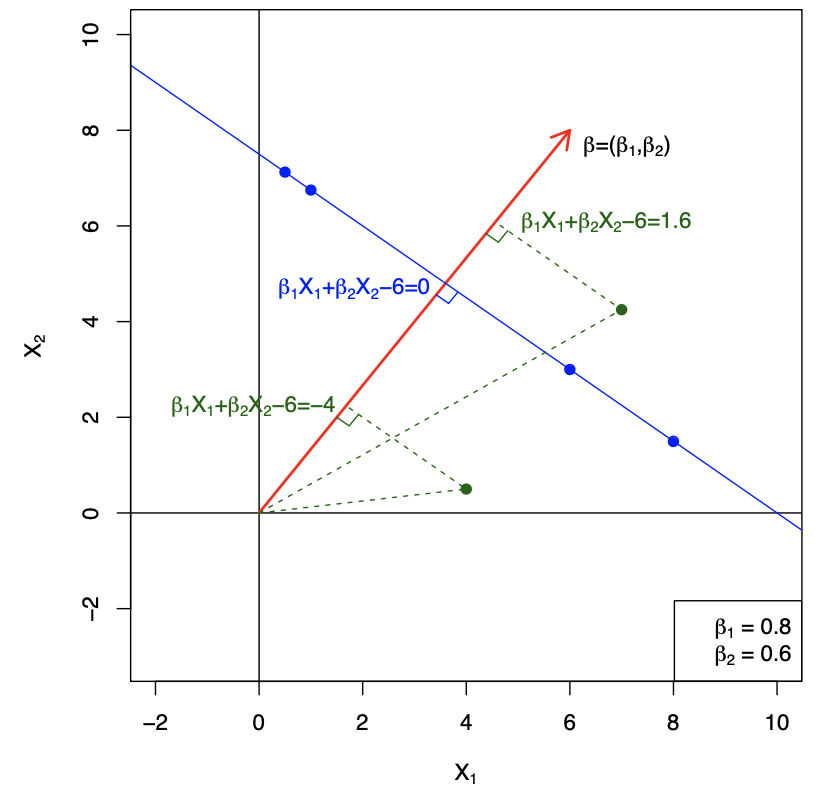## Support Vector Machines

• Here we approach the two-class classification problem in a direct way:

• We try and find a plane that separates the classes in feature space
• If we cannot, we get creative in two ways

• We soften what we mean by “separates”

• We enrich and enlarge the feature space so that separation is possible

## What is a Hyperplane?

• A hyperplane in $$p$$ dimensions is a flat affine subspace of dimension $$p − 1$$

• In general the equation for a hyperplane has a form:

• $$\beta_0 + \beta_1X_1 + \beta_2X_2 + ... + \beta_pX_p= 0$$
• In $$p=2$$ dimensions a hyperplane is a line

• If $$\beta_0=0$$, the hyperplane goes through the origin, otherwise not

• The vector $$\beta = (\beta_1, \beta_2, ..., \beta_p)$$ is called the normal vector

• It points in a direction orthogonal to the surface of a hyperplane
• Hyperplane in 2 Dimensions